# Aqueous Magnetic Fluids Instabilities in a Hele-Shaw Cell

2510 words (10 pages) Essay

1st Feb 2018 Physics Reference this

Tags:

Disclaimer: This work has been submitted by a university student. This is not an example of the work produced by our Essay Writing Service. You can view samples of our professional work here.

Any opinions, findings, conclusions or recommendations expressed in this material are those of the authors and do not necessarily reflect the views of UKEssays.com.

M. RÄ‚CUCIU

“Lucian Blaga” University, Faculty of Science, Dr.Ratiu Street, No.5-7, Sibiu, 550024, Romania

In this study, it was investigated the interface patterns of two immiscible, viscous fluids into the geometry of a horizontal Hele-Shaw cell, considering that one of the fluids is an aqueous magnetic fluid. In general, the total energy of a magnetic fluid system consists of three components: gravitational, surface, and magnetic. For a magnetic fluid into a horizontal Hele-Shaw cell, the gravitational energy is constant and can be inessential, thus leaving only the surface and magnetic energies. The interface between two immiscible fluids, one of them with a low viscosity and another with a higher viscosity, becomes unstable and starts to deform. Dynamic competition in such confined geometry leads finally to the formation of fingering patterns of a magnetic fluid in a Hele-Shaw cell. The computational study upon the interface instabilities patterns in aqueous magnetic fluids was accomplished evaluating fractal dimension in finger-type instabilities. Between the fractal dimension and experimental instability generation time a correlation was established.

(Received January 30, 2009; accepted February 13, 2009)

Keywords: Magnetic fluid, Hele-Shaw cell, Viscous finger instability

1. Introduction

Magnetic fluids are composed of magnetic, around 10 nm, single-domain particles coated with a molecular surfactant and suspended in a carrier liquid . Magnetic fluids confined within Hele-Shaw cell exhibit interesting interfacial instabilities, like fingering instability.

The patterns and shapes formation by diverse physical, chemical and biological systems in the natural world has long been a source of fascination for scientists . Interface dynamics plays a major role in pattern formation. Viscous fingering occurs in the flow of two immiscible, viscous fluids between the plates of a Hele-Shaw cell.

A magnetic fluid is considered paramagnetic because the individual nanoparticle magnetizations are randomly oriented, even the solid magnetic nanoparticles are ferromagnetic . Due to Brownian motion, the thermal agitation keeps the magnetic nanoparticles suspended and the coating prevents the nanoparticles from adhering to each other. In ionic magnetic fluids coating the magnetic nanoparticles are replaced one an other by electrostatic repulsion. Because the magnetic nanoparticles are much smaller than the Hele-Shaw cell thickness, it may neglect their particulate properties and it may consider a continuous paramagnetic fluid.

In this study, there was investigated the interface patterns of two immiscible, viscous fluids into the geometry of a horizontal Hele-Shaw cell, considering that one of the fluids is an aqueous magnetic fluid.

In general, the total energy of a magnetic fluid system consists of three components: gravitational, surface, and magnetic. For a magnetic fluid into a horizontal Hele-Shaw cell, the gravitational energy is constant and can be inessential, thus leaving only the surface and magnetic energies.

The interface between two immiscible fluids, one of them with a low viscosity and another with a higher viscosity, becomes unstable and starts to deform. Dynamic competition in such confined geometry leads finally to the formation of fingering patterns of a magnetic fluid in a two-dimensional geometry (Hele-Shaw cell) [4-5].

Due to pressure gradients or gravity, the separation interface of the two immiscible, viscous fluids undergoes on to Saffman-Taylor instability  and develops finger-like structures. The Saffman-Taylor instability is a widely studied example of hydrodynamic pattern formation where interfacial instabilities evolve.

The computational study upon the interface instabilities patterns in aqueous magnetic fluids was carried out by evaluating the fractal dimension in finger-type instabilities.

2. Experimental

Aqueous magnetic fluids used in this study have been prepared in our laboratory, by applying the chemical precipitation method. In table I are presented the aqueous magnetic fluid samples used in this experimental study.

Table 1. The magnetic fluid samples used in this study (d – physical diameter of the magnetic nanoparticles, constituents of the magnetic fluid samples).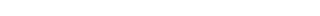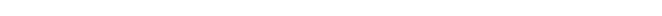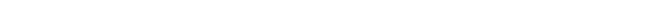Aqueous magnetic fluids instabilities in a Hele-Shaw cell

In this paper, it studies the interface between two immiscible fluids and with different viscosities, one of them with a low viscosity and with magnetic properties (aqueous magnetic fluid) and another with a higher viscosity and non-magnetic (65% aqueous glycerin), when the instability pattern diameter increased in time, instability structure became more and more complex. The splitting of the main finger streamer was occurred in time, determining the irregularity increasing. For each instant image obtained was computed the fractal dimension.

a less viscous fluid is injected into a more viscous one in a 2D geometry (Hele Shaw cell).

Fig. 1 shows a Hele-Shaw cell, used in this experiment, with its two plates of plastic separated by cover microscope slides placed in each corner of the cell, having the same thickness about 300 micrometers. The used fluids are injected through the center hole.

Fig. 2. The finger instability dynamics for aqueous magnetic liquid stabilized with citric acid (A2 sample).

Between the fractal dimension and experimental instability generation time a linear correlations were established for all magnetic fluid samples used in this study (correlation coefficient, R2, 0.962). In Fig. 3 is presented the dynamics of the fractal dimension during the surface image recording, for A1 magnetic fluid sample.

Fig. 1. Hele- Shaw cell used in this experimental study.

In the experiment firstly was injected aqueous glycerin through the central hole to fill the cell. After the cell was filled with glycerin, the magnetic fluid was injected. The surface image recordings were made with a digital camera.

The computational study upon the interface instabilities patterns between aqueous magnetic fluids and glycerin was accomplished evaluating fractal dimension in finger-type instabilities. The fractal analysis was carried out using the box-counting method  as a computational algorithm. In order to apply box-counting method the surface images were analyzed following the HarFA 5.0 software steps, computing the fractal dimension of the each image.

Fig. 3. The dynamics of the fractal dimension in the A1 magnetic fluid sample case, stabilized with

3. Results and discussion

The computational study was accomplished on three sets of images, for aqueous magnetic fluids analyzed in this study, representing finger instabilities developed between two immiscible fluids and with different viscosities.

In Fig. 2 are presented the finger instability dynamics for aqueous magnetic liquid stabilized with citric acid (A2 sample), having a radial symmetry between the fluids injected in the Hele-Shaw cell (glycerin and magnetic fluid).From images shown in Fig. 2 it may be observed that tetrametilamoniu hydroxide.

Also, between the fractal dimension and physical diameter of the magnetic nanoparticles, constituents of the magnetic fluid sample, a correlation was established (a linear regression with a correlation coefficient, R2 = 0.926).

In Fig. 4 it may be observed that for increasing physical diameter of the magnetic nanoparticles was obtained a decreased fractal dimension, after a flow time of magnetic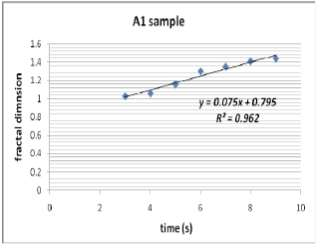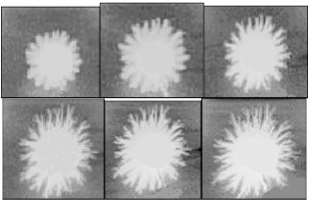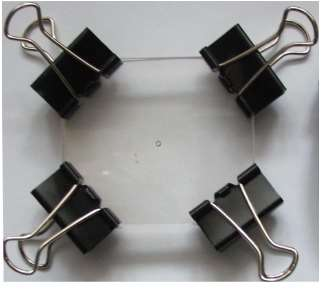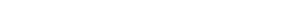fluids about 9 seconds.

134

Fig. 4. Graphical dependence of fractal dimension in function of physical diameter of the magnetic nanoparticles, constituents of the magnetic fluid samples analyzed in this study.

M. RÄƒcuciu

within the Hele-Shaw cell about 7 seconds.

In the Saffman-Taylor instability, if a forward bump is formed on the interface between the fluids, it enhances the pressure gradient and the local interface velocity. Because the velocity of a point on the interface is proportional to the local pressure gradient, the bump grows faster than other parts on the interface. On the other hand, the effect of surface tension competes with this diffusive instability.

Surface tension operates to reduce the pressure at highly curved parts of an interface, and sharp bumps are forced back. Thus, as a result we have the formation of the viscous finger instabilities.

The fractal dimension based analysis proposed in this paper of the aqueous magnetic fluid instability is intended to lead to further mathematical modeling of finger instabilities patterns focused on non-linearity of the magnetic fluid-non-magnetic fluid interface stability.

A linear correlation (correlation coefficient, R2, 0.988) was established between the fractal dimension and viscosity value of the magnetic fluid samples used In Fig. 5 it may be observed that for increased viscosity value of the magnetic fluid was observed increasing the fractal dimension of the interface fluids instability structure.

Fig. 5. Linear correlation between fractal dimension and magnetic fluid viscosity value.

Table 2. Correlation coefficient and standard deviation to the fractal dimension calculation after a flow time of magnetic fluids within the Hele-Shaw cell about 7 seconds.

4. Conclusions

In this paper it was investigated the flow of two immiscible, viscous fluids in the confined geometry of a Hele-Shaw cell.

It may conclude that the fractal dimension values of the finger instabilities pattern images are direct proportional with the magnetic fluid viscosity value and instability generation time, while with the physical diameter of the magnetic nanoparticles constituents of the magnetic fluid a linear negative dependence was evidenced.

The next theoretical analysis step will follow the developing of a convenient model to describe the non-magnetic fluid influence on magnetic fluid surface stability.

References

 P. S. Stevens, Patterns in Nature, Little Brown, Boston (1974).

 S.S. Papel, Low viscosity magnetic fluid obtained by the colloidal suspension of magnetic particles. US Patent 3, 215, 572 (1965).

 R. E. Rosensweig, Ferrohydrodynamics, Cambridge University Press, Cambridge (1985).

 C. Tang, Rev. Mod. Phys., 58, 977 (1986).

 D. Bensimon, L. P. Kadanoff, S. Liang, B. I. Shraiman, C. Tang, Rev.Mod. Phys., 58, 977 (1986).

 P. G. Saffman, G. I. Taylor, Proc. R. Soc. London, Ser. A, 245, 312 (1958).

 T. Tel, A. Fulop, T. Vicsek, Determination of Fractal Dimension for Geometrical Multifractals, Physica A, 159, 155-166 (1989).

________________________

*

In Table 2 the fractal dimension value, correlation coefficient and standard deviation to the fractal dimension calculation, for all magnetic fluid samples used in this experimental study after a flow time of magnetic fluids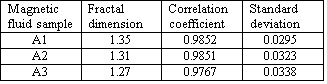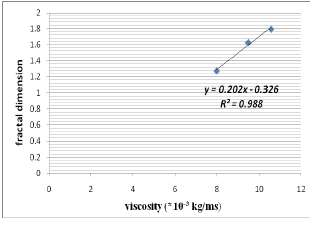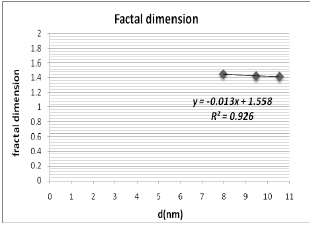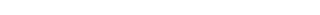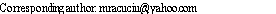## Related Services

View all

### DMCA / Removal Request

If you are the original writer of this essay and no longer wish to have your work published on the UKDiss.com website then please:

Related Services